Next: Computing the Delaunay complex Up: Voronoi Diagram and Delaunay Previous: What is Voronoi diagram   Contents

## What is the Delaunay triangulation in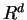?

See also 3.2, 3.1.

Let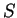be a set of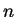points in. The convex hull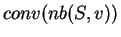of the nearest neighbor set of a Voronoi vertex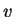is called the Delaunay cell of. The Delaunay complex (or triangulation) ofis a partition of the convex hull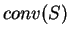into the Delaunay cells of Voronoi vertices together with their faces.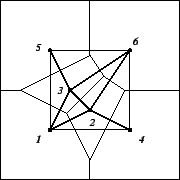The Delaunay complex is not in general a triangulation but becomes a triangulation when the input points are in general position (or nondegenerate), i.e. no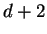points are cospherical or equivalently there is no point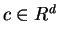whose nearest neighbor set has more than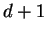elements.

The Delaunay complex is dual to the Voronoi diagram 3.2 in the sense that there is a natural bijection between the two complexes which reverses the face inclusions.

There is a direct way to represent the Delaunay complex, just like the Voronoi diagram 3.2. In fact, it uses the same paraboloid in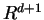: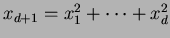. Let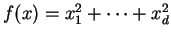, and let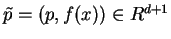for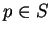. Then the so-called lower hull of the lifted points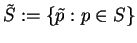represents the Delaunay complex. More precisely, let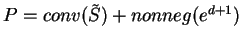where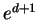is the unit vector inwhose last component is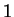. Thus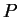is the unbounded convex polyhedron consisting of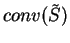and any nonnegative shifts by the upper'' direction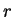. The nontrivial claim is that the the boundary complex ofprojects to the Delaunay complex: any facet ofwhich is not parallel to the vertical directionis a Delaunay cell once its last coordinate is ignored, and any Delaunay cell is represented this way.Next: Computing the Delaunay complex Up: Voronoi Diagram and Delaunay Previous: What is Voronoi diagram   Contents
Komei Fukuda 2004-08-26